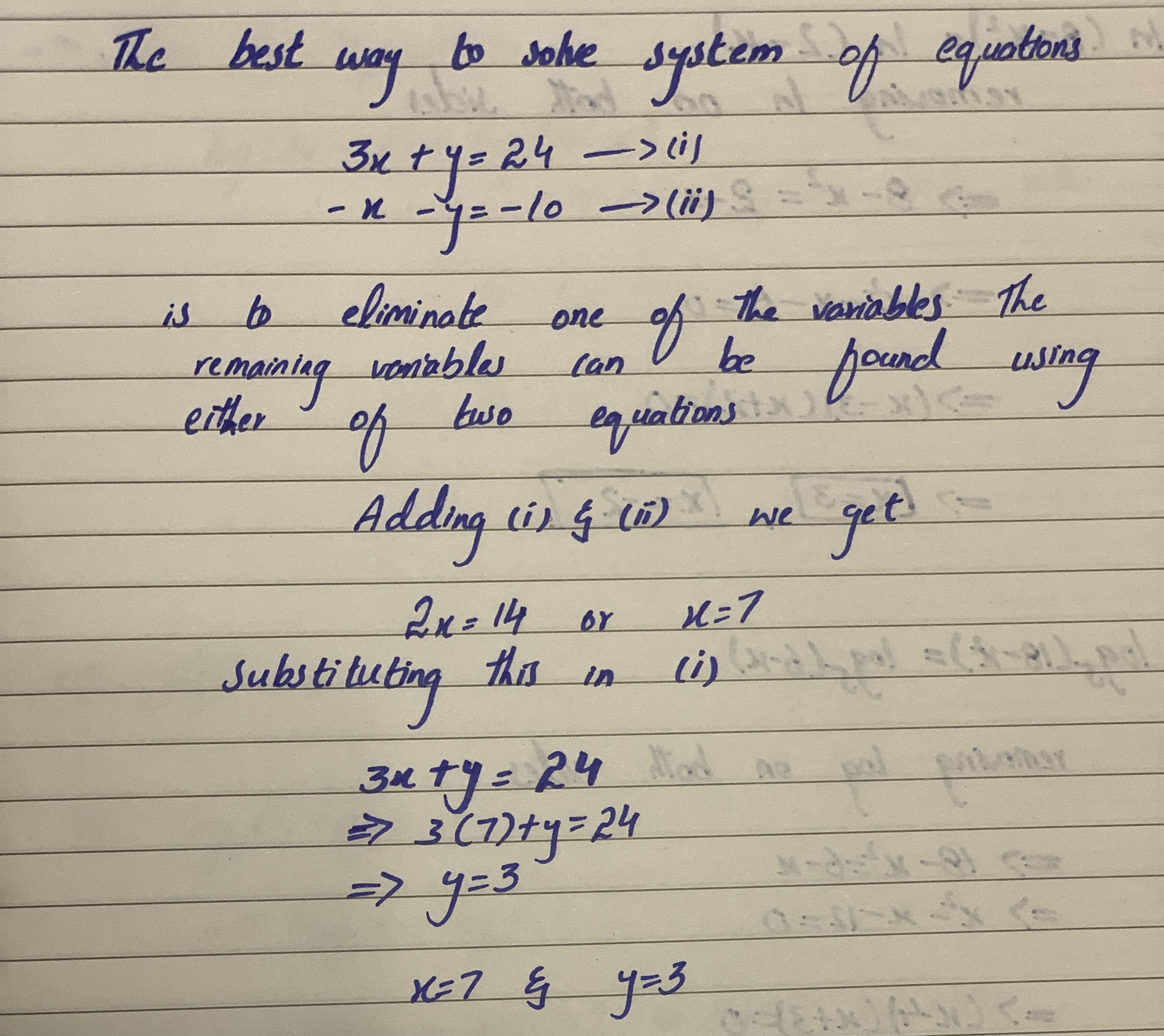Question# For each systems of equations below choose the best method for solving and solve 3x+y=24 -x-y=-10

Equations and inequalities
ANSWEREDFor each systems of equations below choose the best method for solving and solve
3x+y=24
-x-y=-10...........